# Symmetric design

(diff) ← Older revision | Latest revision (diff) | Newer revision → (diff)

symmetric block design

A-design (or balanced incomplete block design, cf. BIBD) which satisfies Fisher's inequality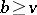(cf. also Block design) with equality. More precisely, a symmetric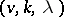-design is an incidence structure consisting ofpoints and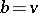blocks (cf. also Block design) of size(that is,-subsets of the point set), such that any two distinct points are on preciselycommon blocks. It can be shown that then also any two distinct blocks intersect in preciselypoints, see [a1], § II.3.

The outstanding problem in this area (1998) is to characterize the possible parameter triples. A simple counting argument gives the equation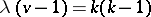, which provides a trivial necessary existence condition. A non-trivial restriction is the Bruck–Ryser–Chowla theorem, see Block design. This condition is not sufficient, as the non-existence of a symmetric-design, i.e. a projective plane of order, shows; see [a5].

There are more thanknown infinite series of symmetric designs (as of 1998). The most classical examples are the symmetric designsformed by the points and hyperplanes of the-dimensional finite projective spaceover the Galois field of order(sois a prime power here) and the Hadamard-designs (or Hadamard configurations) with parameters of the form; such a design is equivalent to a Hadamard matrix of order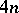, which is conjectured to exist for all values of. Similarly, a symmetric design with parameters of the form(a1)

is equivalent to a regular Hadamard matrix of order, that is, a Hadamard matrix with constant row and column sums; these are conjectured to exist for all values of. Many examples are known, e.g. wheneveris the product of a perfect square with an arbitrary number of the form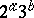. An important recent (1998) construction method for symmetric designs which combines Abelian difference sets (cf. Abelian difference set; Difference set; Difference set (update)) with so-called balanced generalized weighing matrices yields seven infinite families; this is due to Y.J. Ionin [a3]. See [a1], [a2] for lists of the known (1998) infinite series, tables of small symmetric designs and some constructions.

In general, a symmetric design cannot be characterized just by its parameters. For instance, the number of non-isomorphic designs with the same parameters asgrows exponentially with a growth rate of at least, where. Hence it is desirable to characterize the designs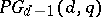as well as other particularly interesting designs. For instance, the Dembowski–Wagner theorem states that a symmetric design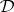with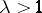in which every line (that is, the intersection of all blocks through two given points) meets every block is isomorphic to some; the same conclusion holds ifadmits an automorphism group which is transitive on ordered triples of non-collinear points. See [a1], § XII.2, for proofs and further characterizations.

Symmetric designs with a "nice" automorphism group are of particular interest. The orbit theorem states that any automorphism groupof a symmetric designhas equally many orbits on points and blocks (cf. also Orbit). In particular,is transitive (cf. Transitive group) on points if and only if it is transitive on blocks. In this case, the permutation rank ofon points agrees with that on blocks (cf. also Rank of a group). In the special case wherehas rank(and thus is doubly transitive on both points and blocks), a complete classification was given by W.M. Kantor [a4]: There are two infinite series, namely the classical designsand another series which has parameters of the form (a1), whereis a power of; the latter examples all admit a symplectic group as automorphism group. In addition, there are two sporadic examples, namely the Hadamard-design on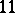points and a-design which admits the Higman–Sims group, one of the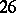sporadic simple groups (cf. also Sporadic simple group). On the other end of the spectrum, there is the case of permutation rank, i.e.is regular (cf. Permutation group) on both points and blocks; such a group is called a Singer group of, generalizing the notion of a Singer cycle. In this case,is equivalent to a-difference setin(cf. also Difference set; Abelian difference set): Up to isomorphism,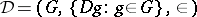. A complete classification is yet (1998) out of reach, even in the Abelian case. For instance, it is widely conjectured that a projective plane of finite orderadmitting a Singer group must be Desarguesian (cf. also Desargues geometry), at least in the cyclic case; but only for a few values of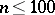, this is actually proven. However, there is ample evidence for the validity of the weaker prime power conjecture, which asserts that the orderof a finite projective plane admitting an Abelian Singer group must be a prime power; this is now known to hold whenever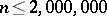. See [a1], § VI.7, where this topic is studied in the language of planar difference sets.

How to Cite This Entry:
Symmetric design. Encyclopedia of Mathematics. URL: http://encyclopediaofmath.org/index.php?title=Symmetric_design&oldid=12425
This article was adapted from an original article by D. Jungnickel (originator), which appeared in Encyclopedia of Mathematics - ISBN 1402006098. See original article